# AP Calculus BC Practice Test 26

### Test Information10 questions20 minutes

Calculator Disallowed

1. A function f (x) equals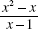for all x except x = 1. For the function to be continuous at x = 1, the value of f (1) must be

2.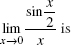3. The first four terms of the Taylor series about x = 0 of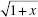are

4. Using the line tangent to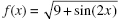at x = 0, an estimate of f (0.06) is

5. Air is escaping from a balloon at a rate of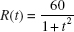cubic feet per minute, where t is measured in minutes. How much air, in cubic feet, escapes during the first minute?

6. The motion of a particle in a plane is given by the pair of equations x = cos 2t, y = sin 2t. The magnitude of its acceleration at any time t equals

7. Let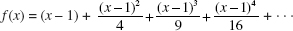The interval of convergence of f (x) is

8. A point moves along the curve y = x2 + 1 so that the x-coordinate is increasing at the constant rate of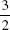units per second. The rate, in units per second, at which the distance from the originis changing when the point has coordinates (1,2) is equal to

9.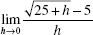10.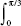sec2 x tan2 x dx equals